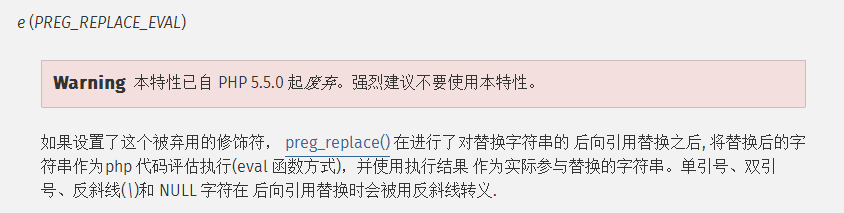# php新版本废弃 preg_replace /e 修饰符

preg_replace(): The /e modifier is deprecated, use preg_replace_callback insteadpreg_replace("/([A-Z])/e", "'_' . strtolower('\\1')", $str) 修改成 preg_replace("/([A-Z])/",'gwyy',$str);
function gwyy($match） { return '_'.strtolower($match);
}

preg_replace("/([A-Z])/e", "'_' . strtolower('\\1')", $str) 修改成 preg_replace_callback('/([A-Z])/', function ($matches) {
return '_' . strtolower($matches); },$str)

class a {

private $joinStr = "__AAAAA__"; public function __construct() {$this->joinStr = preg_replace_callback("/__([A-Z_-]+)__/sU",array($this,'gwyy'),$this->joinStr);
echo  $this->joinStr; } public function gwyy($match) {
print_r($match); return 'aaa'; } }$a = new a();

$patterns = '/'.$begin.$parseTag.$n1.'\/(\s*?)'.$end.'/eis';$replacement    = "\$this->parseXmlTag('$tagLib','$tag','$1','')";
$content = preg_replace($patterns, $replacement,$content);

$that =$this;
$patterns = '/'.$begin.$parseTag.$n1.'\/(\s*?)'.$end.'/is';$content=preg_replace_callback($patterns, function($matches) use($tagLib,$tag,$that){ return$that->parseXmlTag($tagLib,$tag,$matches,''); },$content);©️2019 CSDN 皮肤主题: 编程工作室 设计师: CSDN官方博客